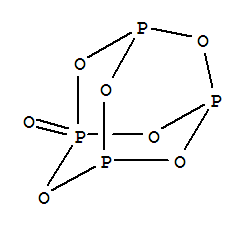# Question 12162

Mar 3, 2017

There are 17 lone pairs in one molecule of ${\text{P"_4"O}}_{7}$.

#### Explanation:

The structure of tetraphosphorus heptoxide, ${\text{P"_4"O}}_{7}$, isEach $\text{O}$ atom has 2 lone pairs, and each single-bonded $\text{P}$ atom has 1 lone pair.

Thus

$\text{Number of lone pairs}$

= 7 color(red)(cancel(color(black)("O atoms"))) × "2 lone pairs"/(1 color(red)(cancel(color(black)("O atom")))) + 3 color(red)(cancel(color(black)("P atoms"))) × "1 lone pair"/(1 color(red)(cancel(color(black)("P atom")))) = "17 lone pairs"#